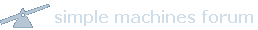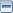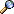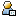Bitcoin ForumFebruary 21, 2020, 04:15:09 AMNews: Latest Bitcoin Core release: 0.19.0.1 [Torrent]Home Help Search Login Register MoreShow Posts Pages: 1 ... 8 9 10 11 12 13 14 15 16 17 18 19 20 21 22 23 24 25 26 27 28 29 30 31 32 33 34 35 36 37 38 39 40 41 42 43 44 45 46 47 48 49 50 51 52 53 54 55 56 57 
 1141 Bitcoin / Bitcoin Discussion / Re: Bitcoin mobile. on: July 17, 2010, 05:09:37 AM Quote from: jago25_98 on June 26, 2010, 07:56:59 PMI think this idea deserves more recognition. A phone is much more accessible and widely available than a computer. There are many, many people for whom they only got a phone. Even some kind of SMS / gateway / key exchange would be something... being able to send a payment by sms to a gateway server with an unsupported phone to a supported phone, for example, or to a trusted third party who can handle the transaction for us. By rolling passcode perhaps. That would fantastic, and possibly not too much of a major overhaul needed?Inspired?I am working on an open source SMS project that may be capable of providing this capability.  However, I am not so familiar with how payment would be handled by text messages.
 1142 Economy / Marketplace / Re: Buying bitcoins, wild-west style on: July 17, 2010, 05:04:49 AM How would it be possible to use SMS to buy/sell bitcoins?
 1143 Bitcoin / Development & Technical Discussion / Calculate Hash Target and Probability in PHP on: July 17, 2010, 04:37:09 AM I am trying to prepare PHP code to calculate exact value for Hash Target similar as to displayed at http://www.alloscomp.com/bitcoin/calculator.php (currently: 1.48501965484E+65).Can someone help me to refine my code?http://jsonrpcphp.org/?page=download&lang=enCalculate current hash target:Code:getdifficulty()); //     \$a      /         \$b // (2^256 - 1) / (2^32 * difficulty) bcscale(256); \$a = bcsub(bcpow(2,256),1); //\$a = gmp_strval(gmp_sub(gmp_pow(2,256),1)); \$b = bcmul(bcpow(2,32),\$difficulty); //\$b = pow(2,32) * \$difficulty; bcscale(0); \$target = bcdiv(\$a,\$b); //\$target = gmp_strval(gmp_div(\$a,\$b)); //\$target = bcdiv(\$a,\$b); //\$target = "148504231478890412392775945444335243545681910455595839046778120430"; //\$target = "148504231478000000000000000000000000000000000000000000000000000000"; //\$target = "148501965484000000000000000000000000000000000000000000000000000000"; //000000000168fd00000000000000000000000000000000000000000000000000 //         168fcfffffee48119ddbfdc811138960d70605cc300000000000000 \$targethex = gmp_strval(gmp_sub(\$target,0),16); echo "Current Hash Target: Dec(\$target)    Hex(\$targethex)";?>Calculate probability:Code:
 1144 Bitcoin / Development & Technical Discussion / SMS/Text Messaging Integration? on: July 17, 2010, 12:42:19 AM is there any usefulness for SMS/Text Messaging integration with Bitcoin in any way?
 1145 Bitcoin / Development & Technical Discussion / Re: Some Statistics on: July 16, 2010, 05:48:00 AM If you have a local ap (apache+php) server, you can run this code and keep it open in browser window and it should update approximately every second.  It isn't 100% precise, but fairly close.Added: last=\$(date +%s); tail -F debug.log | grep --line-buffered height= | sed -u 's/.*\(height=\(.*\)\)/\2/g' | while read line; do now=\$(date +%s); delta=\$(( \$now - \$last)); echo \$now \$delta \$line; last=\$now; done
 1146 Bitcoin / Development & Technical Discussion / Some Statistics on: July 16, 2010, 05:24:25 AM http://nullvoid.org/bitcoin/statistix.phphttp://nullvoid.org/bitcoin/statistix.php?showallblocks (if you want to see timestamps for all 69,000+ blocks until now) (2.5Mb+ output)http://jsonrpcphp.org/?page=download&lang=enstatistix.phpCode:getblockcount(); \$now = date("U"); \$blockfile = "blockdata"; \$data = file(\$blockfile, FILE_IGNORE_NEW_LINES | FILE_SKIP_EMPTY_LINES); array_pop(\$data); foreach (\$data as \$line) { \$blocks = strtok(\$line, " "); \$date = strtok(" "); \$avghash = strtok(" "); }?>
PHP Source Code            |\n";	echo "|---------------------------------------|\n";	echo "|   Last block discovered in duration   |\n";	echo "|       and when it was discovered      |\n";	echo "|---------------------------------------|\n";	echo "|          Now  \$now              |\n";	\$lastdate = \$now - \$date; \$lastdate .= (\$lastdate == 1) ? " sec" : " secs"; \$lastdate .= str_repeat(" ", 10 - strlen(\$lastdate));	\$lastblock = trim(\$blocks); \$lastblock .= str_repeat(" ", 12 - strlen(\$lastblock));	echo "|   Last Block  \$lastdate:\$lastblock |\n";	\$block = array();	foreach (\$data as \$line) {		\$blocks = strtok(\$line, " ");		\$date = strtok(" ");		\$avghash = strtok(" ");		\$block[\$blocks] = \$date;		if ((\$now - 60) <= \$date) { \$min01date = \$date; \$min01blocks = \$blocks; }		if ((\$now - 120) <= \$date) { \$min02date = \$date; \$min02blocks = \$blocks; }		if ((\$now - 180) <= \$date) { \$min03date = \$date; \$min03blocks = \$blocks; }		if ((\$now - 240) <= \$date) { \$min04date = \$date; \$min04blocks = \$blocks; }		if ((\$now - 300) <= \$date) { \$min05date = \$date; \$min05blocks = \$blocks; }		if ((\$now - 600) <= \$date) { \$min10date = \$date; \$min10blocks = \$blocks; }		if ((\$now - 1200) <= \$date) { \$min20date = \$date; \$min20blocks = \$blocks; }		if ((\$now - 1800) <= \$date) { \$min30date = \$date; \$min30blocks = \$blocks; }		if ((\$now - 2400) <= \$date) { \$min40date = \$date; \$min40blocks = \$blocks; }		if ((\$now - 3000) <= \$date) { \$min50date = \$date; \$min50blocks = \$blocks; }		if ((\$now - 3600) <= \$date) { \$hr01date = \$date; \$hr01blocks = \$blocks; }		if ((\$now - 7200) <= \$date) { \$hr02date = \$date; \$hr02blocks = \$blocks; }		if ((\$now - 10800) <= \$date) { \$hr03date = \$date; \$hr03blocks = \$blocks; }		if ((\$now - 14400) <= \$date) { \$hr04date = \$date; \$hr04blocks = \$blocks; }		if ((\$now - 18000) <= \$date) { \$hr05date = \$date; \$hr05blocks = \$blocks; }		if ((\$now - 21600) <= \$date) { \$hr06date = \$date; \$hr06blocks = \$blocks; }		if ((\$now - 51200) <= \$date) { \$hr12date = \$date; \$hr12blocks = \$blocks; }		if ((\$now - 102400) <= \$date) { \$day1date = \$date; \$day1blocks = \$blocks; }		if ((\$now - 204800) <= \$date) { \$day2date = \$date; \$day2blocks = \$blocks; }		if ((\$now - 307200) <= \$date) { \$day3date = \$date; \$day3blocks = \$blocks; }		if ((\$now - 409600) <= \$date) { \$day4date = \$date; \$day4blocks = \$blocks; }		if ((\$now - 512000) <= \$date) { \$day5date = \$date; \$day5blocks = \$blocks; }		if ((\$now - 614400) <= \$date) { \$day6date = \$date; \$day6blocks = \$blocks; }		if ((\$now - 716800) <= \$date) { \$wk01date = \$date; \$wk01blocks = \$blocks; }		if ((\$now - 1433600) <= \$date) { \$wk02date = \$date; \$wk02blocks = \$blocks; }		if ((\$now - 2150400) <= \$date) { \$wk03date = \$date; \$wk03blocks = \$blocks; }		if ((\$now - 2867200) <= \$date) { \$wk04date = \$date; \$wk04blocks = \$blocks; }		if ((\$now - 5734400) <= \$date) { \$wk08date = \$date; \$wk08blocks = \$blocks; }		if ((\$now - 11468800) <= \$date) { \$wk16date = \$date; \$wk16blocks = \$blocks; }		if ((\$now - 22937600) <= \$date) { \$wk32date = \$date; \$wk32blocks = \$blocks; }		if ((\$now - 37376000) <= \$date) { \$yr1date = \$date; \$yr1blocks = \$blocks; }	}	if (\$min01blocks == "") \$min01 = ""; else \$min01 = "\$min01date:\$min01blocks";	if (\$min02blocks == "") \$min02 = ""; else \$min02 = "\$min02date:\$min02blocks";	if (\$min03blocks == "") \$min03 = ""; else \$min03 = "\$min03date:\$min03blocks";	if (\$min04blocks == "") \$min04 = ""; else \$min04 = "\$min04date:\$min04blocks";	if (\$min05blocks == "") \$min05 = ""; else \$min05 = "\$min05date:\$min05blocks";	if (\$min10blocks == "") \$min10 = ""; else \$min10 = "\$min10date:\$min10blocks";	if (\$min20blocks == "") \$min20 = ""; else \$min20 = "\$min20date:\$min20blocks";	if (\$min30blocks == "") \$min30 = ""; else \$min30 = "\$min30date:\$min30blocks";	if (\$min40blocks == "") \$min40 = ""; else \$min40 = "\$min40date:\$min40blocks";	if (\$min50blocks == "") \$min50 = ""; else \$min50 = "\$min50date:\$min50blocks";	if (\$hr01blocks == "") \$hr01 = ""; else \$hr01 = "\$hr01date:\$hr01blocks";	if (\$hr02blocks == "") \$hr02 = ""; else \$hr02 = "\$hr02date:\$hr02blocks";	if (\$hr03blocks == "") \$hr03 = ""; else \$hr03 = "\$hr03date:\$hr03blocks";	if (\$hr04blocks == "") \$hr04 = ""; else \$hr04 = "\$hr04date:\$hr04blocks";	if (\$hr05blocks == "") \$hr05 = ""; else \$hr05 = "\$hr05date:\$hr05blocks";	if (\$hr06blocks == "") \$hr06 = ""; else \$hr06 = "\$hr06date:\$hr06blocks";	if (\$hr12blocks == "") \$hr12 = ""; else \$hr12 = "\$hr12date:\$hr12blocks";	if (\$day1blocks == "") \$day1 = ""; else \$day1 = "\$day1date:\$day1blocks";	if (\$day2blocks == "") \$day2 = ""; else \$day2 = "\$day2date:\$day2blocks";	if (\$day3blocks == "") \$day3 = ""; else \$day3 = "\$day3date:\$day3blocks";	if (\$day4blocks == "") \$day4 = ""; else \$day4 = "\$day4date:\$day4blocks";	if (\$day5blocks == "") \$day5 = ""; else \$day5 = "\$day5date:\$day5blocks";	if (\$day6blocks == "") \$day6 = ""; else \$day6 = "\$day6date:\$day6blocks";	if (\$wk01blocks == "") \$wk01 = ""; else \$wk01 = "\$wk01date:\$wk01blocks";	if (\$wk02blocks == "") \$wk02 = ""; else \$wk02 = "\$wk02date:\$wk02blocks";	if (\$wk03blocks == "") \$wk03 = ""; else \$wk03 = "\$wk03date:\$wk03blocks";	if (\$wk04blocks == "") \$wk04 = ""; else \$wk04 = "\$wk04date:\$wk04blocks";	if (\$wk08blocks == "") \$wk08 = ""; else \$wk08 = "\$wk08date:\$wk08blocks";	if (\$wk16blocks == "") \$wk16 = ""; else \$wk16 = "\$wk16date:\$wk16blocks";	if (\$wk32blocks == "") \$wk32 = ""; else \$wk32 = "\$wk32date:\$wk32blocks";	if (\$yr1blocks == "") \$yr1 = ""; else \$yr1 = "\$yr1date:\$yr1blocks";	echo "|    1min  ago  ".\$min01.str_repeat(" ", 24 - strlen(\$min01))."|\n";	echo "|    2min  ago  ".\$min02.str_repeat(" ", 24 - strlen(\$min02))."|\n";	echo "|    3min  ago  ".\$min03.str_repeat(" ", 24 - strlen(\$min03))."|\n";	echo "|    4min  ago  ".\$min04.str_repeat(" ", 24 - strlen(\$min04))."|\n";	echo "|    5min  ago  ".\$min05.str_repeat(" ", 24 - strlen(\$min05))."|\n";	echo "|   10min  ago  ".\$min10.str_repeat(" ", 24 - strlen(\$min10))."|\n";	echo "|   20min  ago  ".\$min20.str_repeat(" ", 24 - strlen(\$min20))."|\n";	echo "|   30min  ago  ".\$min30.str_repeat(" ", 24 - strlen(\$min30))."|\n";	echo "|   40min  ago  ".\$min40.str_repeat(" ", 24 - strlen(\$min40))."|\n";	echo "|   50min  ago  ".\$min50.str_repeat(" ", 24 - strlen(\$min50))."|\n";	echo "|    1hr   ago  ".\$hr01.str_repeat(" ", 24 - strlen(\$hr01))."|\n";	echo "|    2hrs  ago  ".\$hr02.str_repeat(" ", 24 - strlen(\$hr02))."|\n";	echo "|    3hrs  ago  ".\$hr03.str_repeat(" ", 24 - strlen(\$hr03))."|\n";	echo "|    4hrs  ago  ".\$hr04.str_repeat(" ", 24 - strlen(\$hr04))."|\n";	echo "|    5hrs  ago  ".\$hr05.str_repeat(" ", 24 - strlen(\$hr05))."|\n";	echo "|    6hrs  ago  ".\$hr06.str_repeat(" ", 24 - strlen(\$hr06))."|\n";	echo "|   12hrs  ago  ".\$hr12.str_repeat(" ", 24 - strlen(\$hr12))."|\n";	echo "|    1day  ago  ".\$day1.str_repeat(" ", 24 - strlen(\$day1))."|\n";	echo "|    2days ago  ".\$day2.str_repeat(" ", 24 - strlen(\$day2))."|\n";	echo "|    3days ago  ".\$day3.str_repeat(" ", 24 - strlen(\$day3))."|\n";	echo "|    4days ago  ".\$day4.str_repeat(" ", 24 - strlen(\$day4))."|\n";	echo "|    5days ago  ".\$day5.str_repeat(" ", 24 - strlen(\$day5))."|\n";	echo "|    6days ago  ".\$day6.str_repeat(" ", 24 - strlen(\$day6))."|\n";	echo "|    1wk   ago  ".\$wk01.str_repeat(" ", 24 - strlen(\$wk01))."|\n";	echo "|    2wks  ago  ".\$wk02.str_repeat(" ", 24 - strlen(\$wk02))."|\n";	echo "|    3wks  ago  ".\$wk03.str_repeat(" ", 24 - strlen(\$wk03))."|\n";	echo "|    4wks  ago  ".\$wk04.str_repeat(" ", 24 - strlen(\$wk04))."|\n";	echo "|    8wks  ago  ".\$wk08.str_repeat(" ", 24 - strlen(\$wk08))."|\n";	echo "|   16wks  ago  ".\$wk16.str_repeat(" ", 24 - strlen(\$wk16))."|\n";	echo "|   32wks  ago  ".\$wk32.str_repeat(" ", 24 - strlen(\$wk32))."|\n";	echo "|    1yr   ago  ".\$yr1.str_repeat(" ", 24 - strlen(\$yr1))."|\n";	echo "|---------------------------------------/\n";	if (isset(\$min01blocks)) \$min01c = (\$blockcount - \$min01blocks); else \$min01c = 0; echo "| ".fill(number_format(\$min01c, 0, ".", ","),9)." blocks in last minute\n";	if (isset(\$min02blocks)) \$min02c = (\$blockcount - \$min02blocks); else \$min02c = 0; echo "| ".fill(number_format(\$min02c, 0, ".", ","),9)." blocks in last 2 mins\n";	if (isset(\$min03blocks)) \$min03c = (\$blockcount - \$min03blocks); else \$min03c = 0; echo "| ".fill(number_format(\$min03c, 0, ".", ","),9)." blocks in last 3 mins\n";	if (isset(\$min04blocks)) \$min04c = (\$blockcount - \$min04blocks); else \$min04c = 0; echo "| ".fill(number_format(\$min04c, 0, ".", ","),9)." blocks in last 4 mins\n";	if (isset(\$min05blocks)) \$min05c = (\$blockcount - \$min05blocks); else \$min05c = 0; echo "| ".fill(number_format(\$min05c, 0, ".", ","),9)." blocks in last 5 mins\n";	if (isset(\$min10blocks)) \$min10c = (\$blockcount - \$min10blocks); else \$min10c = 0; echo "| ".fill(number_format(\$min10c, 0, ".", ","),9)." blocks in last 10 mins\n";	if (isset(\$min20blocks)) \$min20c = (\$blockcount - \$min20blocks); else \$min20c = 0; echo "| ".fill(number_format(\$min20c, 0, ".", ","),9)." blocks in last 20 mins\n";	if (isset(\$min30blocks)) \$min30c = (\$blockcount - \$min30blocks); else \$min30c = 0; echo "| ".fill(number_format(\$min30c, 0, ".", ","),9)." blocks in last 30 mins\n";	if (isset(\$min40blocks)) \$min40c = (\$blockcount - \$min40blocks); else \$min40c = 0; echo "| ".fill(number_format(\$min40c, 0, ".", ","),9)." blocks in last 40 mins\n";	if (isset(\$min50blocks)) \$min50c = (\$blockcount - \$min50blocks); else \$min50c = 0; echo "| ".fill(number_format(\$min50c, 0, ".", ","),9)." blocks in last 50 mins\n";	if (isset(\$hr01blocks)) \$hr01c = (\$blockcount - \$hr01blocks); else \$hr01c = 0; echo "| ".fill(number_format(\$hr01c, 0, ".", ","),9)." blocks in last hour\n";	if (isset(\$hr02blocks)) \$hr02c = (\$blockcount - \$hr02blocks); else \$hr02c = 0; echo "| ".fill(number_format(\$hr02c, 0, ".", ","),9)." blocks in last 2 hrs";echo "    blocks/hr avg: ".(\$hr02c / 2)."\n";	if (isset(\$hr03blocks)) \$hr03c = (\$blockcount - \$hr03blocks); else \$hr03c = 0; echo "| ".fill(number_format(\$hr03c, 0, ".", ","),9)." blocks in last 3 hrs";echo "    blocks/hr avg: ".(\$hr03c / 3)."\n";	if (isset(\$hr04blocks)) \$hr04c = (\$blockcount - \$hr04blocks); else \$hr04c = 0; echo "| ".fill(number_format(\$hr04c, 0, ".", ","),9)." blocks in last 4 hrs";echo "    blocks/hr avg: ".(\$hr04c / 4)."\n";	if (isset(\$hr05blocks)) \$hr05c = (\$blockcount - \$hr05blocks); else \$hr05c = 0; echo "| ".fill(number_format(\$hr05c, 0, ".", ","),9)." blocks in last 5 hrs";echo "    blocks/hr avg: ".(\$hr05c / 5)."\n";	if (isset(\$hr06blocks)) \$hr06c = (\$blockcount - \$hr06blocks); else \$hr06c = 0; echo "| ".fill(number_format(\$hr06c, 0, ".", ","),9)." blocks in last 6 hrs";echo "    blocks/hr avg: ".(\$hr06c / 6)."\n";	if (isset(\$hr12blocks)) \$hr12c = (\$blockcount - \$hr12blocks); else \$hr12c = 0; echo "| ".fill(number_format(\$hr12c, 0, ".", ","),9)." blocks in last 12 hrs";echo "   blocks/hr avg: ".(\$hr12c / 12)."\n";	if (isset(\$day1blocks)) \$day1c = (\$blockcount - \$day1blocks); else \$day1c = 0; echo "| ".fill(number_format(\$day1c, 0, ".", ","),9)." blocks in last day";echo "      blocks/hr avg: ".(\$day1c / 24)."\n";	if (isset(\$day2blocks)) \$day2c = (\$blockcount - \$day2blocks); else \$day2c = 0; echo "| ".fill(number_format(\$day2c, 0, ".", ","),9)." blocks in last 2 days";echo "   blocks/hr avg: ".(\$day2c / 48)."\n";	if (isset(\$day3blocks)) \$day3c = (\$blockcount - \$day3blocks); else \$day3c = 0; echo "| ".fill(number_format(\$day3c, 0, ".", ","),9)." blocks in last 3 days";echo "   blocks/hr avg: ".(\$day3c / 72)."\n";	if (isset(\$day4blocks)) \$day4c = (\$blockcount - \$day4blocks); else \$day4c = 0; echo "| ".fill(number_format(\$day4c, 0, ".", ","),9)." blocks in last 4 days";echo "   blocks/hr avg: ".(\$day4c / 96)."\n";	if (isset(\$day5blocks)) \$day5c = (\$blockcount - \$day5blocks); else \$day5c = 0; echo "| ".fill(number_format(\$day5c, 0, ".", ","),9)." blocks in last 5 days";echo "   blocks/hr avg: ".(\$day5c / 120)."\n";	if (isset(\$day6blocks)) \$day6c = (\$blockcount - \$day6blocks); else \$day6c = 0; echo "| ".fill(number_format(\$day6c, 0, ".", ","),9)." blocks in last 6 days";echo "   blocks/hr avg: ".(\$day6c / 144)."\n";	if (isset(\$wk01blocks)) \$wk01c = (\$blockcount - \$wk01blocks); else \$wk01c = 0; echo "| ".fill(number_format(\$wk01c, 0, ".", ","),9)." blocks in last week";echo "     blocks/hr avg: ".(\$wk01c / 168)."\n";	if (isset(\$wk02blocks)) \$wk02c = (\$blockcount - \$wk02blocks); else \$wk02c = 0; echo "| ".fill(number_format(\$wk02c, 0, ".", ","),9)." blocks in last 2 wks";echo "    blocks/hr avg: ".(\$wk02c / 336)."\n";	if (isset(\$wk03blocks)) \$wk03c = (\$blockcount - \$wk03blocks); else \$wk03c = 0; echo "| ".fill(number_format(\$wk03c, 0, ".", ","),9)." blocks in last 3 wks";echo "    blocks/hr avg: ".(\$wk03c / 504)."\n";	if (isset(\$wk04blocks)) \$wk04c = (\$blockcount - \$wk04blocks); else \$wk04c = 0; echo "| ".fill(number_format(\$wk04c, 0, ".", ","),9)." blocks in last 4 wks";echo "    blocks/hr avg: ".(\$wk04c / 672)."\n";	if (isset(\$wk08blocks)) \$wk08c = (\$blockcount - \$wk08blocks); else \$wk08c = 0; echo "| ".fill(number_format(\$wk08c, 0, ".", ","),9)." blocks in last 8 wks";echo "    blocks/hr avg: ".(\$wk08c / 1344)."\n";	if (isset(\$wk16blocks)) \$wk16c = (\$blockcount - \$wk16blocks); else \$wk16c = 0; echo "| ".fill(number_format(\$wk16c, 0, ".", ","),9)." blocks in last 16 wks";echo "   blocks/hr avg: ".(\$wk16c / 2688)."\n";	if (isset(\$wk32blocks)) \$wk32c = (\$blockcount - \$wk32blocks); else \$wk32c = 0; echo "| ".fill(number_format(\$wk32c, 0, ".", ","),9)." blocks in last 32 wks";echo "   blocks/hr avg: ".(\$wk32c / 5376)."\n";	if (isset(\$yr1blocks)) \$yr1c = (\$blockcount - \$yr1blocks); else \$yr1c = 0; echo "| ".fill(number_format(\$yr1c, 0, ".", ","),9)." blocks in last year";echo "     blocks/hr avg: ".(\$yr1c / 8760)."\n";	echo "\----------------------------------------";	if (isset(\$_GET["showallblocks"])) {		echo "\n\n";		foreach (\$block as \$key => \$num) {			\$secs = \$block[\$key] - \$block[\$key-1];			\$secs .= (\$secs == 1) ? " second " : " seconds";			\$secs = str_repeat(" ", 14 - strlen(\$secs)).\$secs;			if (isset(\$block[\$key-1])) echo "\$secs to find block \$key\n";			else echo "  unknown time to find block \$key\n";		}	} else {		echo "\\\n   Timestamp data for all ".number_format(\$blockcount)." blocks  |\n";		echo "                 ~".number_format(filesize(\$blockfile) * 1.23175 / 1024 / 1024, 2)."Mb                 |\n";	echo "-----------------------------------------/";	}	function fill(\$str, \$len) { return str_repeat(" ", \$len - strlen(\$str)).\$str; }?>
Additionally, "blockdata" is updated every minute using a cron script written in python.  This python code populates "blockdata" with " "courtesy of ArtForzgetblockdata.pyCode:#!/usr/bin/env pythonimport structimport hashlibdef uint256_deser(s): r = 0L for i in xrange(8): t = struct.unpack(">= 32 return rsdef uint256_from_compact(c): nbytes = (c >> 24) & 0xFF v = (c & 0xFFFFFFL) << (8 * (nbytes - 3)) return vdef get_difficulty(c): return float(uint256_from_compact(0x1D00FFFF) * 1000 // uint256_from_compact(c)) / 1000.class CBlock: def deserialize(self, s): self.nVersion = struct.unpack(" uint256_from_compact(self.nBits): raise ValueError("bad hash in %s" % repr(self)) self.next = [] self.blocknum = -1 def __repr__(self): return "CBlock{ver=%08x hp=%064x hm=%064x nt=%08x nb=%08x nn=%08x h=%064x, n=%i}" % (self.nVersion, self.hashPrevBlock, self.hashMerkleRoot, self.nTime, self.nBits, self.nNonce, self.hash, self.blocknum)def get_chain_len(blk): r = 1 while len(blk.next) == 1: blk = blk.next r += 1 if len(blk.next) > 1: bestchainlen = 0 for nextblk in blk.next: chainlen = get_chain_len(nextblk) if chainlen > bestchainlen: bestchainlen = chainlen r += bestchainlen return rdef readblocks(filename): f = open(filename, "rb") blocks = [] idxmap = {} while True: try: magic = f.read(4) if magic != "\xf9\xbe\xb4\xd9": break blklen = struct.unpack(" 1: bestnextblk = None bestchainlen = 0 for nextblk in blk.next: chainlen = get_chain_len(nextblk) if chainlen > bestchainlen: bestchainlen = chainlen bestnextblk = nextblk elif chainlen == bestchainlen: if nextblk.nTime < bestnextblk.nTime: bestchainlen = chainlen bestnextblk = nextblk blk.next = [bestnextblk] blk.next = blk.next curblkidx += 1 blk = blk.next blk = rootblk while blk: #print "%i %i %.3f 0x%08X" % (blk.blocknum, blk.nTime, get_difficulty(blk.nBits), blk.nBits) avghashes = 2**256 / uint256_from_compact(blk.nBits) print "%i %i %i" % (blk.blocknum, blk.nTime, avghashes) blk = blk.nextif __name__ == "__main__": print readblocks("/path/to/blk0001.dat")line for cronCode:*/1     *       *       *       *       /path/to/getblockdata.py > /path/to/blockdata
 1147 Bitcoin / Bitcoin Discussion / Seeking Funding or Hosting on: July 15, 2010, 05:48:07 AM I'm recently unemployed and have since established much interest in Bitcoin community as well as contributing developmental aspects towards some related things, but I need a job to survive/support myself.  Is anyone hiring?  :3
 1148 Bitcoin / Bitcoin Discussion / Re: Flood attack 0.00000001 BC on: July 15, 2010, 05:41:07 AM Quote from: gavinandresen on July 12, 2010, 12:08:45 PMFrom the source code:Code:main.h:        // To limit dust spam, require a 0.01 fee if any output is less than 0.01I added this little tidbit into my IRC bot:According to http://is.gd/dsqcH the world population is 6,855,986,675. If everyone had the same amount of bitcoins, everyone would have 0.00306302 bitcoins.  If 0.00000001 bitcoins were equivalent to US\$0.01, then everyone would have equivalent of US\$3,063.01645489 and the value of all bitcoins would be equivalent of US\$21,000,000,000,000.00.How would the BTC0.01 fee affect things considering above?
 1149 Bitcoin / Bitcoin Discussion / Re: Official Bitcoin Unicode Character? on: July 15, 2010, 05:16:30 AM A B with two lines through it without the circle may be a bit confusing or too similar to the Thai currency considering the US\$ symbol is sometimes used with one or two lines.  So additionally a circle around it like the icon used with the client and also the favicon used at this site seems like a good idea.
 1150 Bitcoin / Bitcoin Discussion / Re: Lost all my Bitcoins? on: July 15, 2010, 03:45:26 AM A tiny bit now, but in a couple years?  Here's a snippet from my IRC bot.According to http://www.census.gov/main/www/popclock.html the world population is 6,855,970,029. If everyone had the same amount of bitcoins, everyone would have 0.00306302 bitcoins.  If 0.00000001 bitcoins were equivalent to US\$0.01, then everyone would have equivalent of US\$3,063.02389176 and the value of all bitcoins would be equivalent of US\$21,000,000,000,000.00.
 1151 Bitcoin / Bitcoin Discussion / Official Bitcoin Unicode Character? on: July 15, 2010, 03:02:28 AM I suggest a character representative of symbol for Bitcoin currency be submitted to http://unicode.org/pending/proposals.html฿ doesn't seem to be worthy considering it already is well established as Thai baht (currency).
 Pages: 1 ... 8 9 10 11 12 13 14 15 16 17 18 19 20 21 22 23 24 25 26 27 28 29 30 31 32 33 34 35 36 37 38 39 40 41 42 43 44 45 46 47 48 49 50 51 52 53 54 55 56 57 
Sponsored by , a Bitcoin-accepting VPN.